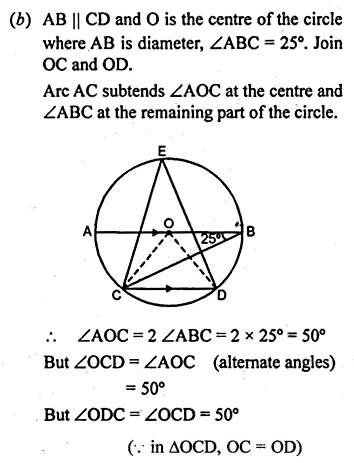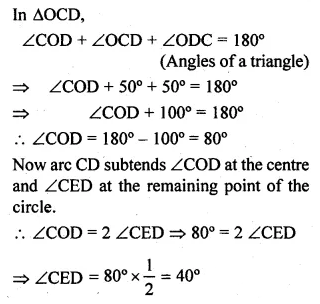Guru

# Question 13. (a) In the figure (i) given below, AB is a diameter of a circle with centre O. AC and BD are perpendiculars on a line PQ. BD meets the circle at E. Prove that AC = ED. (b) In the figure (ii) given below, O is the centre of a circle. Chord CD is parallel to the diameter AB. If ∠ABC = 25°, calculate ∠CED.

• 0

This is an important and exam oriented question from Chapter name- circles
Topic – Angle properties of circles
Chapter number- 15(

a)we have been given in the figure AB is a diameter of a circle with centre O. AC and BD are perpendiculars on a line PQ. BD meets the circle at E.  Now we have to Prove that AC = ED.

(b) In the figure (ii) given below, O is the centre of a circle. Chord CD is parallel to the diameter AB. If ∠ABC = 25°,  now we have to calculate ∠CED.

ICSE Avichal publication
Understanding ICSE Mathematics
Question 13

Share